# Train a Machine Learning Model with Jupyter Notebook

Jupyter is a common web-based notebook for users to interactively write python programs together with documents. In our demo Spark cluster template, Jupyter has been pre-configured to connect to the Spark cluster.

In the following, we show how to use Jupyter to run a small machine job on Spark cluster interactively. Particularly, we’ll use Spark ML library to create a model to predict customer churn in a telecommunication company.

## 1. Open the Jupyter notebook.

Connect web browser to http://<masterIP>:8888 or click on the Jupyter Notebook link in the cluster info page as shown below.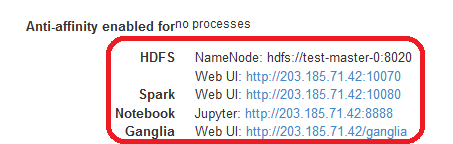Then, users will see the home page of Jupyter notebook few examples.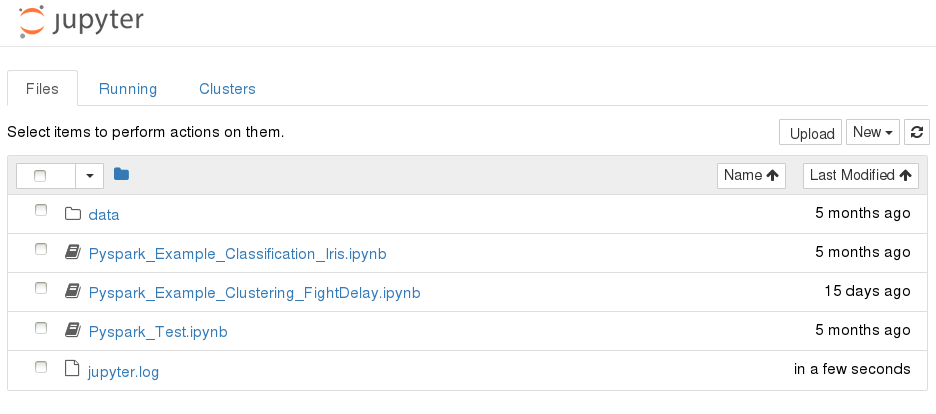Users can create a new notebook, upload a notebook, or open a shell console.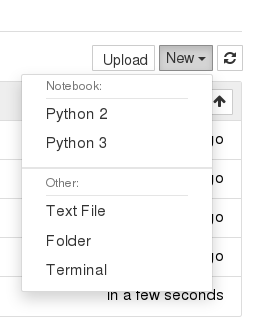## 2. Download and copy sample dataset to HDFS.

First, open a shell console. Click New -> Terminal on the Jupyter home page.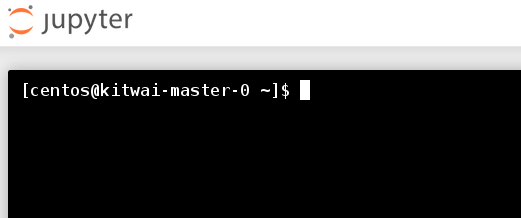Then, execute the following shell commands.

``````wget https://github.com/kitwaicloud/kitwaicloud.github.io/raw/master/dataset/churn-bigml-80.csv
wget https://github.com/kitwaicloud/kitwaicloud.github.io/raw/master/dataset/churn-bigml-20.csv
hdfs dfs -mkdir dataset
hdfs dfs -put churn-bigml-80.csv dataset
hdfs dfs -put churn-bigml-20.csv dataset
``````

Check the sample dataset in HDFS

``````hdfs dfs -ls dataset
Found 2 items
-rw-r--r--   1 centos supergroup      56329 2018-03-13 17:22 dataset/churn-bigml-20.csv
-rw-r--r--   1 centos supergroup     223998 2018-03-13 09:39 dataset/churn-bigml-80.csv

``````

This dataset provides details of customers and labels if they cancel the subscription.

## 3. Create a new Python 3 notebook

Click on New -> Python 3.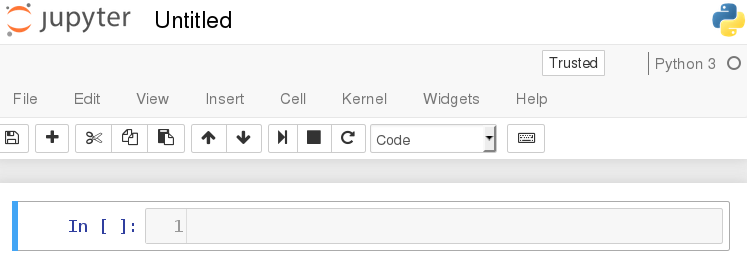Then, we can start writing python code into Jupyter code cells. For each code cell, we can run it.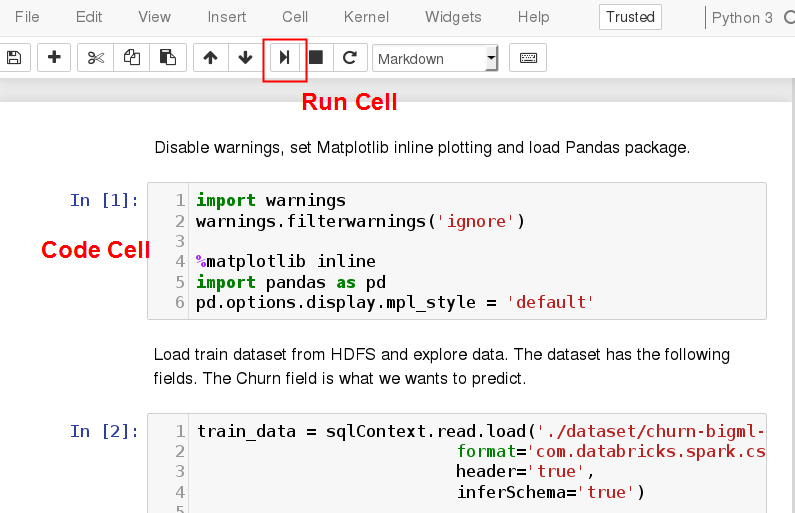Next, we will train a machine learning model by a series of code blocks. Code and run each of them sequentially to gain interactive programming experience. Otherwise, the entire notebook can be downloaded from https://github.com/kitwaicloud/kitwaicloud.github.io/raw/master/jupyter/ChurnPrediction.ipynb

## 4. Load and explore data

Disable warnings, set Matplotlib inline plotting and load Pandas package.

``````import warnings
warnings.filterwarnings('ignore')

%matplotlib inline
import pandas as pd
#pd.options.display.mpl_style = 'default'    # mpl_style is deprecated
``````

Load train dataset from HDFS and explore data. The dataset has the following fields. The Churn field is what we wants to predict.

``````train_data = sqlContext.read.load('./dataset/churn-bigml-80.csv',
format='com.databricks.spark.csv',
inferSchema='true')

train_data.cache()
train_data.printSchema()
``````

The output show that the dataset consists of 20 fields.

``````root
|-- State: string (nullable = true)
|-- Account length: integer (nullable = true)
|-- Area code: integer (nullable = true)
|-- International plan: string (nullable = true)
|-- Voice mail plan: string (nullable = true)
|-- Number vmail messages: integer (nullable = true)
|-- Total day minutes: double (nullable = true)
|-- Total day calls: integer (nullable = true)
|-- Total day charge: double (nullable = true)
|-- Total eve minutes: double (nullable = true)
|-- Total eve calls: integer (nullable = true)
|-- Total eve charge: double (nullable = true)
|-- Total night minutes: double (nullable = true)
|-- Total night calls: integer (nullable = true)
|-- Total night charge: double (nullable = true)
|-- Total intl minutes: double (nullable = true)
|-- Total intl calls: integer (nullable = true)
|-- Total intl charge: double (nullable = true)
|-- Customer service calls: integer (nullable = true)
|-- Churn: boolean (nullable = true)
``````

Explore the first five rows.

``````pd.DataFrame(train_data.take(5), columns=train_data.columns)
``````
State Account length Area code International plan Voice mail plan Number vmail messages Total day minutes Total day calls Total day charge Total eve minutes Total eve calls Total eve charge Total night minutes Total night calls Total night charge Total intl minutes Total intl calls Total intl charge Customer service calls Churn
0 KS 128 415 No Yes 25 265.1 110 45.07 197.4 99 16.78 244.7 91 11.01 10.0 3 2.70 1 False
1 OH 107 415 No Yes 26 161.6 123 27.47 195.5 103 16.62 254.4 103 11.45 13.7 3 3.70 1 False
2 NJ 137 415 No No 0 243.4 114 41.38 121.2 110 10.30 162.6 104 7.32 12.2 5 3.29 0 False
3 OH 84 408 Yes No 0 299.4 71 50.90 61.9 88 5.26 196.9 89 8.86 6.6 7 1.78 2 False
4 OK 75 415 Yes No 0 166.7 113 28.34 148.3 122 12.61 186.9 121 8.41 10.1 3 2.73 3 False

Show summary statistics of all fields.

``````train_data.describe().toPandas()
``````
summary State Account length Area code International plan Voice mail plan Number vmail messages Total day minutes Total day calls Total day charge Total eve minutes Total eve calls Total eve charge Total night minutes Total night calls Total night charge Total intl minutes Total intl calls Total intl charge Customer service calls
0 count 2666 2666 2666 2666 2666 2666 2666 2666 2666 2666 2666 2666 2666 2666 2666 2666 2666 2666 2666
1 mean None 100.62040510127532 437.43885971492875 None None 8.021755438859715 179.48162040510135 100.31020255063765 30.512404351087813 200.38615903976006 100.02363090772693 17.033072018004518 201.16894223555968 100.10615153788447 9.052689422355604 10.23702175543886 4.467366841710428 2.764489872468112 1.5626406601650413
2 stddev None 39.56397365334985 42.521018019427174 None None 13.61227701829193 54.21035022086982 19.988162186059512 9.215732907163497 50.95151511764598 20.16144511531889 4.330864176799864 50.780323368725206 19.418458551101697 2.2851195129157564 2.7883485770512566 2.4561949030129466 0.7528120531228477 1.3112357589949093
3 min AK 1 408 No No 0 0.0 0 0.0 0.0 0 0.0 43.7 33 1.97 0.0 0 0.0 0
4 max WY 243 510 Yes Yes 50 350.8 160 59.64 363.7 170 30.91 395.0 166 17.77 20.0 20 5.4 9

Count churn customers.

``````train_data.groupBy('Churn').count().show()
``````

The output is as follows.

``````+-----+-----+
|Churn|count|
+-----+-----+
| true|  388|
|false| 2278|
+-----+-----+
``````

## 5. Create a Logistic Regression model

Convert categorical fields into numeric fields.

``````from pyspark.ml.feature import StringIndexer
from pyspark.ml.feature import OneHotEncoder

ipIndexer = StringIndexer(inputCol = 'International plan', outputCol = 'ipIndex')
ipEncoder = OneHotEncoder(inputCol = 'ipIndex', outputCol = 'ipVector')
``````

Define feature fields. We choose to ignore some fields as we think they are not relevant.

``````from pyspark.ml.feature import VectorAssembler

featureCols = ['Account length', 'ipVector', 'Number vmail messages', 'Total day minutes',
'Total day calls', 'Total eve minutes', 'Total eve calls', 'Total night minutes', 'Total night calls',
'Total intl minutes', 'Total intl calls', 'Customer service calls']
assembler = VectorAssembler(inputCols = featureCols, outputCol = 'features')
``````

Create a logistic transformer.

``````from pyspark.ml.classification import LogisticRegression

lr = LogisticRegression(maxIter = 20)
``````

Create numeric label field.

``````from pyspark.sql.types import DoubleType
from pyspark.sql.functions import udf

toNum = udf(lambda v: float(v), DoubleType())
train_data = train_data.withColumn('label', toNum(train_data['Churn'])).cache()
pd.DataFrame(train_data.take(5), columns=train_data.columns)
``````
State Account length Area code International plan Voice mail plan Number vmail messages Total day minutes Total day calls Total day charge Total eve minutes ... Total eve charge Total night minutes Total night calls Total night charge Total intl minutes Total intl calls Total intl charge Customer service calls Churn label
0 KS 128 415 No Yes 25 265.1 110 45.07 197.4 ... 16.78 244.7 91 11.01 10.0 3 2.70 1 False 0.0
1 OH 107 415 No Yes 26 161.6 123 27.47 195.5 ... 16.62 254.4 103 11.45 13.7 3 3.70 1 False 0.0
2 NJ 137 415 No No 0 243.4 114 41.38 121.2 ... 10.30 162.6 104 7.32 12.2 5 3.29 0 False 0.0
3 OH 84 408 Yes No 0 299.4 71 50.90 61.9 ... 5.26 196.9 89 8.86 6.6 7 1.78 2 False 0.0
4 OK 75 415 Yes No 0 166.7 113 28.34 148.3 ... 12.61 186.9 121 8.41 10.1 3 2.73 3 False 0.0

5 rows × 21 columns

Chain all transformers and train the model.

``````from pyspark.ml import Pipeline

pipeline = Pipeline(stages=[ipIndexer, ipEncoder, assembler, lr])
model = pipeline.fit(train_data)
``````

Display auROC of the trained model.

``````lrModel = model.stages[-1]
trainingSummary = lrModel.summary
print ('Train auROC = %3.2f' % trainingSummary.areaUnderROC)
``````
``````Train auROC = 0.81
``````

Plot ROC Curve.

``````import matplotlib.pyplot as plt

roc = trainingSummary.roc.toPandas()
plt.plot(roc['FPR'], roc['TPR'])
plt.ylabel('False Positive Rate')
plt.xlabel('True Positive Rate')
plt.title('ROC Curve')
plt.show()
``````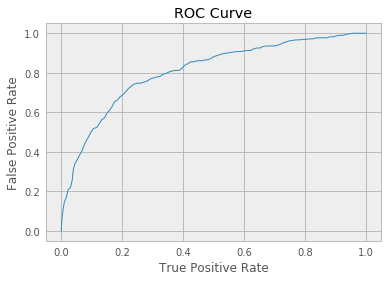## 6. Evaluate the model

Load test dataset from HDFS and evaluate the model with test data.

``````test_data = sqlContext.read.load('./dataset/churn-bigml-20.csv',
format='com.databricks.spark.csv',
inferSchema='true')

test_data.cache()
test_data = test_data.withColumn('label', toNum(test_data['Churn'])).cache()

predictions = model.transform(test_data)
``````

Evaluate the auROC of the test data.

``````from pyspark.ml.evaluation import BinaryClassificationEvaluator

evaluator = BinaryClassificationEvaluator(labelCol='label', rawPredictionCol='rawPrediction')
auROC = evaluator.evaluate(predictions)
print("Test auROC = %3.2f" % auROC)
``````
``````Test auROC = 0.80
``````

Evaluate the accuracy of the test data.

``````from pyspark.ml.evaluation import MulticlassClassificationEvaluator

multi = MulticlassClassificationEvaluator(labelCol='label', predictionCol='prediction', metricName="accuracy")
accuracy = multi.evaluate(predictions)
print("Accuracy = %3.2f" % accuracy)
``````
``````Accuracy = 0.850075
``````

Users can try to adjust training parameters to improve the accuracy of prediction.

## 7. Export the trained model for deployment

Export the model into PMML format. The PMML file can be downloaded and deployed with other tools such as Openscoring. Users can also deploy the PMML model with KitWai Openscoring cluster. See How to Deploy a Spark ML Model for more details.

``````from pyspark2pmml import PMMLBuilder
pmmlBytes = PMMLBuilder(sc, train_data, model).buildByteArray()

# Jpmml sparkml lib for python 2 is no longer supported.
#from jpmml_sparkml import toPMMLBytes
#pmmlBytes = toPMMLBytes(sc, train_data, model)

#print(pmmlBytes.decode("UTF-8"))

pmml = pmmlBytes.decode("UTF-8")
file = open("/tmp/churn.pmml","w")
file.write(pmml)
file.close()
``````